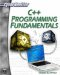# Typedefs

A structure is only one way that you can define your own types of variables in C++, the typedef is another. A typedef in C++ is a type of variable declaration. The purpose of the typedef is to create new types from existing data types. Because a typedef is simply a declaration, it can be intermingled with standard variable declarations. However, it is common practice to declare all typedefs before declaring the standard variables. It is also common practice to capitalize the first letter of a typedef to distinguish it from the built-in types, which all have lowercase names. Typedefs are often used to create synonyms for built-in types.

`typedef int Integer; //The word 'Integer' can now be                    // used in place of int int k,j      // this creates two ints Integer m,n; // this also creates two ints`

In this example, a typedef was used to substitute for a built-in type. This is generally not recommended. Why not just use the built-in type? However, because it is used in code you may see, it is important for you to see this application of typedef. Usually, a typedef associates a type name with a more complicated type specification. Typedefs are often used with arrays. A typedef should always be used in situations where the same type definition is used more than once for the same purpose.

`typedef int myarray; //10 integers myarray a,b; // This creates two arrays int x, y; //This does the same thing without                    //typedef `

Basically, the typedef is a way for you to create your own new labels for data types. You can use it with any data type, even as a synonym for an existing basic type such as int. It is recommended that you only use it with more complex data types such as an array. Typedefs can even be used for other types you create such as structures. Consider the following code sample.

### Example 8.5

Step 1: Type the following code into your favorite text editor.

`#include <cmath> #include <iostream> using namespace std;   typedef double ANSWER; // function prototypes ANSWER squareroot(double number); ANSWER cuberoot(double number); ANSWER fourthroot(double number); int main() {      int menu;      double num;      ANSWER response; cout << "1. Square root \n";      cout << "2. Cube root \n";      cout << "3. Fourth root \n";      cout << "Enter your selection\n";      cin >> menu;      switch (menu)      {      case 1:    cout << "Enter your number \n";      cin >> num;    response = squareroot(num);      break;      case 2:    cout << "Enter your number \n";      cin >> num;    response = cuberoot(num);      break;      case 3:    cout << "Enter your number \n";      cin >> num;    response = fourthroot(num);      break;      default:      cout << "Invalid entry\n";      }      cout << "The answer is " << response;      return 0; } ANSWER squareroot(double number) {  return sqrt(number); } ANSWER cuberoot(double number) {  return pow(number,.3333); } ANSWER fourthroot(double number) {  return pow (number,.25); }`

Step 2: Compile that code.

As you can see, the typdef ANSWER can now be used in any math function that needs to return an answer. This can be useful for you when you have a particular data type that is used in a specialized way in a variety of places. You can also move the typedef to a header file so that you can include it in any program that you wish to use it in.

You should also notice a few other things about this particular example. In addition to illustrating the use of a typedef, it also shows you how to use the pow function in a creative way to get higher roots of numbers. For example, the fourth root of a number is the same as raising that number to the th power, or the .25 power. Although it is not the purpose of this book to teach you math, it is important that you learn to take various principles, combine them, and apply them in creative ways. That type of creative thinking marks the difference between mediocre programmers and great programmers.C++ Programming Fundamentals (Cyberrookies)
ISBN: 1584502371
EAN: 2147483647
Year: 2005
Pages: 197
Authors: Chuck Easttom